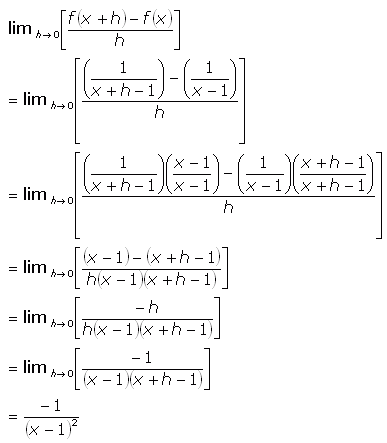SEARCH HOMEMath Central Quandaries & QueriesQuestion from Michelle, a student: im having trouble finding the derivative of f(x)=1/(x-1) using the f(x+h)-f(x)/h method.Michelle,

Just cross-multiply the fractions to create the common denominator and
things fall out nicely.Cheers,
Stephen La Rocque.Math Central is supported by the University of Regina and The Pacific Institute for the Mathematical Sciences.